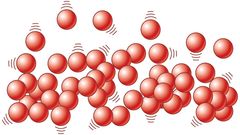Shipping costs € 12 | FREE from € 99

Gastos de envío Península 3,99€
GRATIS a partir de 38€

# The trap of the energy balance

Energy can neither be created nor destroyed, only transformed. Sure you have heard before this statement. And it is true, it is the principle of conservation of energy, that is derived from the first law of thermodynamics:
Energy that enters a system = Energy out of system + Variation of internal energy of the system
This is the basis on which is built the theory of energy balance. The theory of energy balance states that, as energy can neither be created nor destroyed, the calories that enter the human body less calories out (expended), are the calories that will accumulate or lose fat:
Calories in - Calories out = Variation of the fatty tissue
But this is a deduction wrong the first law of thermodynamics. The approach of the energy balance equation it is not compatible with the law of conservation of energy or the first law of thermodynamics.
Let's see why.
The first law of thermodynamics states that the variation of internal energy of a system is equal to the sum of the energy exchanges with the environment. The trap that makes the theory of energy balance is that, in the equation of the first law of thermodynamics, replaces the variation of internal energy of the system (the human body) by the variation of energy stored in the fatty tissue, and is not the same.
The internal energy and the energy stored in the fatty tissue are not equivalent and you can't substitute in the first law of thermodynamics. The equation of energy balance mix churras with merino.
Without going into details, the internal energy of the system this is the sum of the energies of all its particles. Due to its complexity, the internal energy of a body cannot be measured. You can only calculate the difference between 2 states.The theory of energy balance cheats and replaces concepts that are not equivalent.
But, why does it seem that the energy balance works in some cases?
Sure that you know someone is counting calories to lose or gain weight and it works for her. This is so because, in reality, is not applying the energy balance, even if you believe that, yes. The method that follow these people is the following:
1. To establish a hypothesis of energy expenditure of a “maintenance”
2. Define a percentage of “deficit” or “surplus” heat according to the needs
3. Configure the rations of the diet